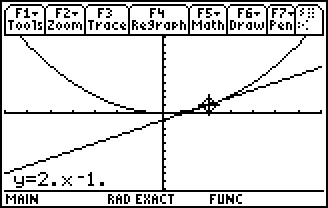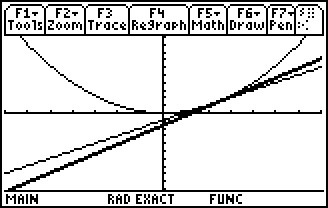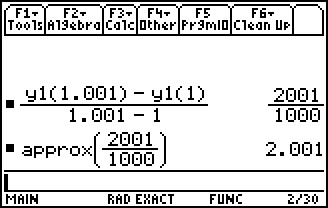# Activities

••• ##### Subject Area

• Math: Calculus: Derivatives

• ##### Author9-12

15 Minutes

• ##### Device
• TI-89 / TI-89 Titanium

## Secant and Tangent Lines#### Activity Overview

In this activity, students will observe the slopes of the secant line and tangent line as point Q on the function approaches the other point P.

#### Key Steps

•In this activity, students will explore graphically, numerically, and algebraically. First, students will graph the function y1(x) = x2 and the tangent to the function at x = 1.

•Students will then graph the secant line and compare it to the tangent line as the slope decreases and the intersection points of the secant line and function curve get closer together.

•Students will then calculate the average rate of change of y1(x) on an interval and use the slope of the secant line to estimate the instantaneous rate of change of y1(x) at a given x value.